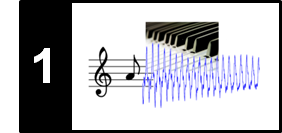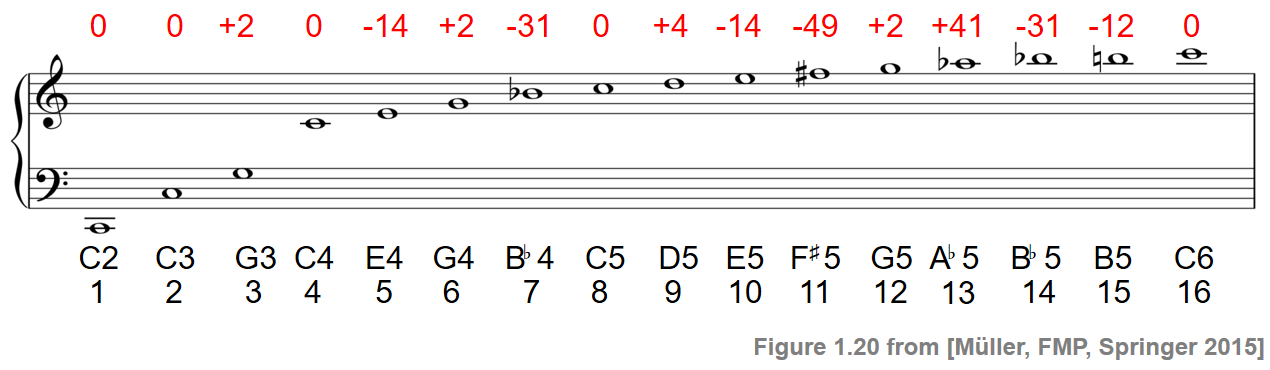# Harmonic Series

In this notebook we have a closer look at the harmonic series following Section 1.3.2 of [Müller, FMP, Springer 2015].

Let $\omega$ denote the center frequency of a musical note. For example, the music note C2 (having MIDI note number $p=36$) has the center frequency $\omega=65.4$ Hz. The harmonic series is an arithmetic series $\omega$, $2\omega$, $3\omega$, $4\omega$, $\ldots$, where the difference between consecutive harmonics is constant and equal to the fundamental frequency. Since our perception of pitch is logarithmic in frequency, we perceive higher harmonics as "closer together" than lower ones. This is different for the octave series, which is defined by the geometric progression $\omega$, $2\omega$, $4\omega$, $8\omega$, and so on. For an octave series, the difference between consecutive frequencies is perceived as "the same" in the sense of musical interval. Consequently, in terms of what we hear, each octave in the harmonic series is divided into increasingly "smaller" and more numerous intervals.

Let us again consider the note C2 ($p=36$) with center frequency $\omega=65.4$ Hz. Then, the second harmonic ($2\omega$) sounds like a C3 (one octave higher), the third harmonic ($3\omega$) like a G3 (a so-called perfect fifth above C3), and the fourth harmonic ($4\omega$) like a C4 (two octaves higher). Starting with a C2, the following figure shows for each of the first $16$ harmonics the musical note that is closest in terms of the difference between the harmonic's frequency and the center frequency of the note as specified by

$$F_\mathrm{pitch}(p) = 2^{(p-69)/12} \cdot 440.$$

Furthermore, the difference (in cents) between each harmonic's frequency and the center frequency of the closest note is indicated (top row, in red).For example, the frequency of the third harmonic is just $2$ cents above the center frequency of G3, which is much smaller than the just noticeable difference. In contrast, the frequency of the $11^{\mathrm{th}}$ harmonic is $49$ cents below the center frequency of the note F5, which is nearly half a semitone and clearly audible. If the harmonics are transposed into the span of one octave (by suitably multiplying or dividing the frequencies by a power of two), they approximate certain notes of the twelve-tone equal-tempered scale. Some of the twelve scale steps are approximated well such as the ones for C ($1^{\mathrm{st}}$ harmonic), G ($3^{\mathrm{rd}}$ harmonic), or D ($9^{\mathrm{th}}$ harmonic), whereas others are problematic such as F$^\sharp$ ($11^{\mathrm{th}}$ harmonic), A$^\flat$ ($13^{\mathrm{th}}$ harmonic), or B$^\flat$ ($7^{\mathrm{th}}$ harmonic).

The following code produces sinusoids for all of the 16 harmonic frequencies and 16 center frequencies of the notes shown in the figure above.

In :
import numpy as np
import matplotlib.pyplot as plt
import IPython.display as ipd
import pandas as pd
from collections import OrderedDict

import sys
sys.path.append('..')
import libfmp.c1

# Computation of frequencies and differences
p = 36
freq = libfmp.c1.f_pitch(p)
freq_harmonic = (np.asarray(range(16)) + 1) * freq
sinusoid_freq_harmonic = []
notes = np.asarray([36, 48, 55, 60, 64, 67, 70, 72, 74, 76, 78, 79, 80, 82, 83, 84])
freq_center = libfmp.c1.f_pitch(notes)
sinusoid_freq_center = []
freq_deviation_cents =  libfmp.c1.difference_cents(freq_harmonic, freq_center)

# Generation of sinusoids
dur = 4  # seconds
Fs = 4000  # sampling rate

for freq in freq_center:
x, t = libfmp.c1.generate_sinusoid(dur=dur, Fs=Fs, freq=freq)
sinusoid_freq_center.append(x)

for freq in freq_harmonic:
x, t = libfmp.c1.generate_sinusoid(dur=dur, Fs=Fs, freq=freq)
sinusoid_freq_harmonic.append(x)

# Generation of html table
audio_tag_html_center = []
for i in range(len(freq_center)):
audio_tag = ipd.Audio(sinusoid_freq_center[i], rate=Fs)
audio_tag_html = audio_tag._repr_html_().replace('\n', '').strip()
audio_tag_html = audio_tag_html.replace('<audio ', '<audio style="width: 100px; "')
audio_tag_html_center.append(audio_tag_html)

audio_tag_html_harmonic = []
for i in range(len(freq_harmonic)):
audio_tag = ipd.Audio(sinusoid_freq_harmonic[i], rate = Fs)
audio_tag_html = audio_tag._repr_html_().replace('\n', '').strip()
audio_tag_html = audio_tag_html.replace('<audio ', '<audio style="width: 100px; "')
audio_tag_html_harmonic.append(audio_tag_html)

pd.set_option('display.max_colwidth', None)
df = pd.DataFrame(OrderedDict([('Note', ['C2', 'C3', 'G3', 'C4', 'E4', 'G4',
'B$^\\flat$4', 'C5', 'D5', 'E5', 'F$^\sharp$5',
'G5', 'A$^\\flat$5', 'B$^\\flat$5', 'B5', 'C6']),
('Note Freq. (Hz)', freq_center),
('Note Sinusoid', audio_tag_html_center),
('Harmonic Freq. (Hz)', freq_harmonic),
('Harmonic Sinusoid', audio_tag_html_harmonic),
('Deviation (Cents)', freq_deviation_cents)]))

df.index = np.arange(1, len(df) + 1)
ipd.HTML(df.to_html(escape=False, float_format='%.2f'))

Out:
Note Note Freq. (Hz) Note Sinusoid Harmonic Freq. (Hz) Harmonic Sinusoid Deviation (Cents)
1 C2 65.41 65.41 0.00
2 C3 130.81 130.81 0.00
3 G3 196.00 196.22 1.96
4 C4 261.63 261.63 0.00
5 E4 329.63 327.03 -13.69
6 G4 392.00 392.44 1.96
7 B$^\flat$4 466.16 457.84 -31.17
8 C5 523.25 523.25 0.00
9 D5 587.33 588.66